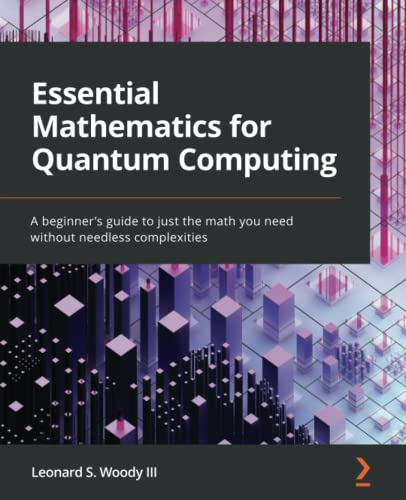# Essential Mathematics for Quantum Computing: A beginner’s guide to just the math you need without needless complexities

• Length: 252 pages
• Edition: 1
• Publisher:
• Publication Date: 2022-04-22
• ISBN-10: 1801073147
• ISBN-13: 9781801073141
Description

Demystify quantum computing by learning the math it is built on

#### Key Features

• Build a solid mathematical foundation to get started with developing powerful quantum solutions
• Understand linear algebra, calculus, matrices, complex numbers, vector spaces, and other concepts essential for quantum computing
• Learn the math needed to understand how quantum algorithms function

#### Book Description

Quantum computing is an exciting subject that offers hope to solve the world’s most complex problems at a quicker pace. It is being used quite widely in different spheres of technology, including cybersecurity, finance, and many more, but its concepts, such as superposition, are often misunderstood because engineers may not know the math to understand them. This book will teach the requisite math concepts in an intuitive way and connect them to principles in quantum computing.

Starting with the most basic of concepts, 2D vectors that are just line segments in space, you’ll move on to tackle matrix multiplication using an instinctive method. Linearity is the major theme throughout the book and since quantum mechanics is a linear theory, you’ll see how they go hand in hand. As you advance, you’ll understand intrinsically what a vector is and how to transform vectors with matrices and operators. You’ll also see how complex numbers make their voices heard and understand the probability behind it all.

It’s all here, in writing you can understand. This is not a stuffy math book with definitions, axioms, theorems, and so on. This book meets you where you’re at and guides you to where you need to be for quantum computing. Already know some of this stuff? No problem! The book is componentized, so you can learn just the parts you want. And with tons of exercises and their answers, you’ll get all the practice you need.

#### What you will learn

• Operate on vectors (qubits) with matrices (gates)
• Define linear combinations and linear independence
• Understand vector spaces and their basis sets
• Rotate, reflect, and project vectors with matrices
• Realize the connection between complex numbers and the Bloch sphere
• Determine whether a matrix is invertible and find its eigenvalues
• Probabilistically determine the measurement of a qubit
• Tie it all together with bra-ket notation

#### Who this book is for

If you want to learn quantum computing but are unsure of the math involved, this book is for you. If you’ve taken high school math, you’ll easily understand the topics covered. And even if you haven’t, the book will give you a refresher on topics such as trigonometry, matrices, and vectors. This book will help you gain the confidence to fully understand quantum computation without losing you in the process!